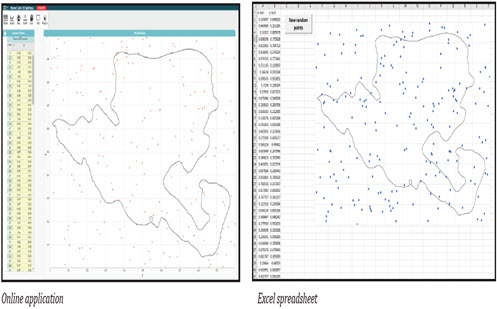Go to website

# reSolve: Monte Carlo Simulations

This sequence of two lessons explores how statistical techniques that rely on randomly generated data can be used to solve problems. In the first lesson, students compare different methods for calculating the area of an irregular shape, using the context of oil spill maps. They are introduced to the Monte Carlo method for statistically calculating area. In the second lesson, they use the Monte Carlo method to estimate the area of a quadrant of a circle. By comparing this with the formula for the area of a circle, they estimate the value of pi (π). The lessons are outlined in detail including curriculum links, vocabulary, sample answers, spreadsheets of data, discussion points and student resources. This sequence is part of the reSolve: Mathematics by Inquiry program.

Year level(s) Year 9
Audience Teacher
Purpose Teaching resource
Teaching strategies and pedagogical approaches Mathematics investigation
Keywords area, central tendency, polygons, statistical frequency, mean, median, pi

## Curriculum alignment

Curriculum connections Numeracy
Strand and focus Statistics, Build understanding, Apply understanding, Measurement
Topics Chance and probability, Data representation and interpretation
AC: Mathematics content descriptions
ACMMG216 Calculate areas of composite shapes
ACMMG222 Investigate Pythagoras’ Theorem and its application to solving simple problems involving right angled triangles
ACMSP226 Calculate relative frequencies from given or collected data to estimate probabilities of events involving 'and' or 'or'
ACMSP283 Compare data displays using mean, median and range to describe and interpret numerical data sets in terms of location (centre) and spread
National numeracy learning progression Understanding geometric properties
Interpreting and representing data - IRD5
Understanding units of measurement - UuM9